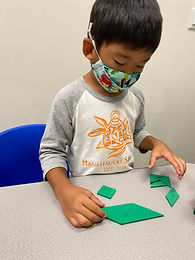## Ms. Brianne

### Target 1​

###### Lesson Type:

Continuation

Number Operation

:

Computation

Multiply within 100.

###### 1:

Understand that multiplication can be represented with arrays, repeated addition, and skip counting.

###### 2:

Understand that the same quantity can be group in multiple ways, creating different multiplication equations.

###### 3:

Understand that multiplication equations can be written both vertically and horizontally and multiplication is often identified by an “x” in an equation.

3rd

###### Vocabulary:

Multiply, Times, Product, Equal groups

Activities:

• Students used repeated addition or skip counting as they solved different multiplication problems in a game of tic tac toe.
• Students solved multiplication problems as they tried to get from the start to finish on a game board.
• Students created various skyscrapers using grid paper to make their own picture of a city skyline. Then they wrote the multiplication equation that matched each buildings' array.### Home Exploration

###### Guiding Questions:## Absent Students:

### Target 2

:

###### 1:

Compose larger shapes from smaller shapes.

###### 2:

Using triangles in a tangram set, make triangles, squares, and parallelograms.

###### 3:

Using all seven pieces in a tangram set, make squares, parallelograms, triangles, trapezoids, and rectangles.

###### 4:

Study geometric shapes to derive the component shapes that can be used to create it.

4th

###### Vocabulary:

2D Shapes, Tangrams, Composite Shape

Activities:

• Students combined tangrams to compose larger shapes.
• Students used all 7 pieces in a tangram set to make triangles, squares and rectangles.### Home Exploration

###### Guiding Questions:### Target 3

:

###### Vocabulary:

Activities:### Home Exploration